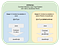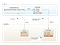# Learn Once Use Everywhere: Using JavaScript APIs in Java for Web Browser

Last time we saw how we can reuse our Java code in JavaScript from my article “Web Browser Programming in Java”. Today we’ll take a look on how we could call the JavaScript APIs, web apps and frameworks from our Java code and at the end to transpile them back to JavaScript so that it will be runnable on the web browser.

To see how we could do this, it is important that we understand the process on how Java with GWT (http://www.gwtproject.org) or J2CL (https://github.com/google/j2cl) communicates with JavaScript. For this purpose we have the JsInterop which work as a bridge between Java and JavaScript. In the old version of GWT we had JSNI (JavaScript Native Interface) but this is now deprecated and we should only use JsInterop. In JsInterop you have two cases:

Use case 1: the contracts are available in Java and you want to use those contracts in JavaScript. This is shown in our Calculator example from my first article. In this case you want to use the Java apps and APIs from your JavaScript apps.

Use case 2: the contracts are available in JavaScript as APIs or apps or frameworks and you want to use them in Java. In this case you need to bridge the JavaScript APIs to your Java apps. At the end you transpile everything back to JavaScript since you want to run your app on the web browser.

Following picture shows both use cases for JsInterop.JsInterop: Contract between Java Type System and Native JavaScript Type System

In this article I will show you how to do the use case 2.

First of all we need to have a JavaScript app or APIs which we want to use in our Java app. For this simple example I would use a JavaScript Calculator which is implemented in the index.html file as a JavaScript function:

`<!doctype html><html><head>    <script type="text/javascript" src="calculator.nocache.js"    </script>    <script type="text/javascript">        Calculator = function () {            this.x;            this.y;        };        Calculator.prototype.sum = function () {            return this.x + this.y;        };    </script></head><body>    ...</body></html>`

The Calculator has just two values x and y. It also has a function sum to calculate the sum of x + y. The next step is how we can bridge this Calculator function into our Java world? It’s quite easy, we just write a JsInterop class Calculator.java to map it to our Java world. We use isNative = true to say to our transpiler that the implementation of this class is in the JavaScript world:

`package com.github.lofi.client;import ...;@JsType(namespace = JsPackage.GLOBAL, name = "Calculator",         isNative = true)public class Calculator {    public int x    public int y;    public native int sum();}`

In the class above you don’t see any implementations of the Calculator since they reside in the JavaScript function. That’s it.

But wait, how can we use this Java class Calculator in our Java web app? For this purpose I extend the HTML file just like following steps:

1. I add the result of the transpiled JavaScript file: calculator.nocache.js to the HTML file
2. In the component Button event onclick we can use the CalculatorService and call the calculatePrice method.
`<!doctype html><html><head>    <script type="text/javascript" src="calculator.nocache.js"    </script>    <script type="text/javascript">        Calculator = function () {            this.x;            this.y;        };        Calculator.prototype.sum = function () {            return this.x + this.y;        };    </script></head><body>    <button onclick="console.log(new        CalculatorService().calculatePrice(41, 2));">Click my            Calculator!    </button></body></html>`

So the Calculator class can be used directly within the CalculatorService class in Java and at the end the CalculatorService class can be called from the JavaScript component Button within onclick event. For this purpose we need to build CalculatorService and this class should be callable from JavaScript which represents the use case 1 above. Here is the implementation of CalculatorService class which uses the Calculator class:

`package com.github.lofi.client;import ...;@JsType(namespace = JsPackage.GLOBAL, name = "CalculatorService")public class CalculatorService {    public String calculatePrice(int x, int y) {        Calculator calculator = new Calculator();        calculator.x = x;        calculator.y = y;        final int sum = calculator.sum();        return "Price: " + sum;    }}`

That’s all we need.

To sum up, in this short article we have seen how we could access JavaScript APIs from Java by creating a JsInterop file which has a “native” implementation. The property “native” means that the implementation lies in JavaScript, which represents the use case 2. In the example above the implementation of the Calculator is done in JavaScript. From CalculatorService we can call the JavaScript APIs easily as they were implemented in Java. In this context I also show you again how we would call the CalculatorService from the JavaScript which shows us the use case 1. Detail information about the use case 1 can be found in my first article.

The diagram below shows the dependencies and interactions between Java and JavaScript for both classes CalculatorService and Calculator.CalculatorService and Calculator — Dependencies and Interactions — Java and JavaScript: diagram created with GenMyModel: http://bit.ly/UMLCalculatorJsInterop

Enjoy and have fun with Java on the Web browser!

After understanding both use cases for JsInterop, next time I’ll show you how to implement web browser persistence with IndexedDB using Elemental2 APIs — only in Java.

All examples can be found at:
https://github.com/lofidewanto/jsinterop-simple-native-example

Padlet for GWT / J2CL modern programming: